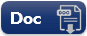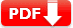# Wonderlic Test QPS SET-2 : Sample Question Answers [PDF]

Wonderlic Test QPS SET-2 : Sample Question Answers [PDF]: This is sample study guide for Wonderlic Cognitive Ability Practice Test 2020. You can either download our Wonderlic Test QPS SET-2 PDF or join in our free quiz.

## Wonderlic Test: 10 Sample Question AnswersQPS SET-2

 1. If three inches of rope cost 7 cents, how much would 2 feet of rope cost? 🔘 56 cents 🔘 66 cents 🔘76 cents 🔘176 cents 🔘 286 cents
Answer: A 56 cents. First, divide the number of inches in 2 feet (24) by 3; then, multiply this number (8) by the price of 3 inches of rope: 8 x 7 = 56.
 2. What is the next number in the sequence: 5, 10, 20, 40,…? 🔘 60 🔘 70 🔘 80 🔘85 🔘 90
Answer: C 80.The next number is found by multiplying the previous number by 2.
 3. What is the next number in the sequence: 3, 8, 18, 38…? 🔘 48 🔘 58 🔘 68 🔘 78 🔘 88
Answer:D 78.The next number is taken by adding one to each number and then multiplying this sum by 2.
 4. What is the next number in the sequence: 5, 9, 17,33,…? 🔘39 🔘 47 🔘 59 🔘65 🔘 77
Answer:D 65. The next number is found by multiplyingthe number by 2 and then subtracting one from theproduct..
 5. What is the next number in the sequence: 9, 3, 1, 1/3,…? 🔘 1/3 🔘1/9 🔘 2/5 🔘2/9 🔘 3/6
 6. What is the next number in the sequence: 24, 12, 6, 3…? 🔘 2 🔘2.5 🔘 1 🔘1.5 🔘 0
6. Answer:C 1.5. The next number is found by dividing each number by 2.
 7. Which of the following numbers represents the greatest amount: 6, 6.0, 0.600, 60? 🔘 6.00 🔘60.0 🔘 60 🔘0.60 🔘 NOTA
7. Answer: C 60.Be sure to identify the position of the decimal point
 8. Which of the following numbers represents the smallest amount: 0.400, 0.04, 4.0, 40? 🔘 40.0 🔘 0.40 🔘 0.4 🔘0.04 🔘 0.400
 Document Type Download Link Free Editable Doc FileFree Printable PDF File